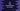# C# program to find the circumference of a circle## C# program to find the circumference of a circle:

In this post, we will learn how to find the circumference of a circle in C#. If the radius of a circle is r and if π is the mathematical constant PI, we can find the circumference of a circle by using the below formula:

``Circumference = 2 * π * radius``

π is a mathematical constant and it’s value is already defined. So, if we can get the value of radius from the user, we can calculate the value of circumference or perimeter for that circle.

### C# program:

Let’s write down the program.

``````using System;

public class Program
{
public static void Main()
{
double circumference;

circumference = 2 * Math.PI * radius;

Console.WriteLine("Circumference :"+Math.Round(circumference, 2));
}
}``````

Here,

• We are calculating the circumference by using the above formula. The value of π is reading as Math.PI, which is the constant defined in the Math class.
• The calculated circumference is stored in the variable circumference.
• The last line is printing the value of circumference.

If you run this program, it will print output as like below:

``````Enter the value of radius:
13
Circumference :81.68``````

### Calculate the circumference by using a separate function:

We can also use a separate function to calculate the circumference. This function will take the radius of the circle as parameter and return the circumference of that circle.

Below is the complete program:

``````using System;

public class Program
{
return 2 * Math.PI * radius;
}

public static void Main()
{
double circumference;